# What's the best way to solve the physics

## Solving physics problems

The approach will now be explained step by step.

First step: Summary of all given sizes in the Base units

time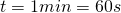speed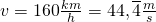Now we solve the individual parts of the task one after the other:

Exercise part a)

Preliminary consideration:

The size we are looking for is the Acceleration a. The acceleration is defined as the change in speed over time.

The definition can therefore be found in the formula collection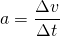.

Both the speed (change in speed) and the time required for it are given, so we can use this in the definition of the acceleration:

1. Needed formula for the size to be calculated (here: a) write down:2. values (in Base units!) deploy and Result write down: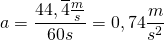.

Note: All units in the formula are recorded. The unity of the result is even more important. A result without a unit is useless!

Note: If you put the base units in the formula, the base unit always comes out! The only meaningful unit of acceleration is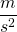!

Always use exact values!

When converting the speed to m / s, you may get a value with an infinite number of decimal places (in this example a numerical value with a period). When inserting, write down the rounded value, expected but will with the exact value. Only when Bottom line will be again rounded (usually two places after the decimal point if there is no zero in front of it).

3. Answer sentence Do not forget (depending on the specifications):

The mean acceleration of the train is.

Exercise part b)

Preliminary consideration:

Now the path or the Route s searched that is covered during acceleration.

The Path-time law of uniformly accelerated motion reads:.

We can plug the required values ​​directly into this formula and get:Here, too, the exact value calculated beforehand should be used for the acceleration. The unit of time must be seconds. Notice the correct spelling: The time with the unit is in brackets, the square applies to the numerical value and the unit!

Answer sentence: The acceleration distance is 1,333.33m.

Exercise part c)

now is the Acceleration time t not known. In order to be able to calculate the path, the unknown quantity must be replaced by a known relationship.

We remember that Velocity-time law of uniformly accelerated motion (the definition of acceleration changed after v). It is said: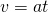.

This formula can be rearranged after t and into the Way-time law deploy. So the formulated solution is:

The following applies:In addition, the following applies: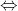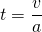Insertion provides: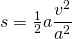a can be shortened, and we write the "2" under the fraction line: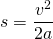Now the values ​​are inserted: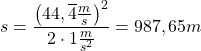Note: The exact (not rounded) value is used again for the speed.

Also note the correct spelling of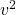: The numerical value with the unit is in brackets, both are squared!

Answer sentence: The acceleration distance is 987.65m.

Note: Another possibility would have been to first calculate the acceleration time t with the aid of the speed-time law and then insert this into the distance-time law. However, the path shown here is a little more elegant and should be known to you in any case. Replacing a size with a known context is an important basic skill that you should master.

Exercise part d)

Now the Time t wanted, in which the train comes to a stop.

The braking acceleration is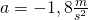.

The following applies to the time (see above):Now the values ​​are inserted: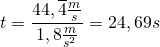[The negative sign has been omitted here, since of course the time cannot be negative. It would be mathematically correct to also assign a negative sign to the speed, because the speedmodification when braking negative is - the speed will smaller!]

Answer: The braking process takes just under 25 seconds.

Control:

After completing each part of the task, it makes sense to check the plausibility of the result. In many cases you can at least estimate the magnitude of the result before the calculation. The acceleration of the train is certainly significantly smaller than the acceleration due to gravity (g = 9.81 m / s2).

If the result is outside the estimated order of magnitude, you should check the calculation again - maybe you have swapped denominator and numerator, forgot a zero, forgot to square a quantity, etc.

Such errors are usually easy to find by looking for them. By complying with the Basic rules however, they can usually be avoided from the outset.

More about the control can be found below.